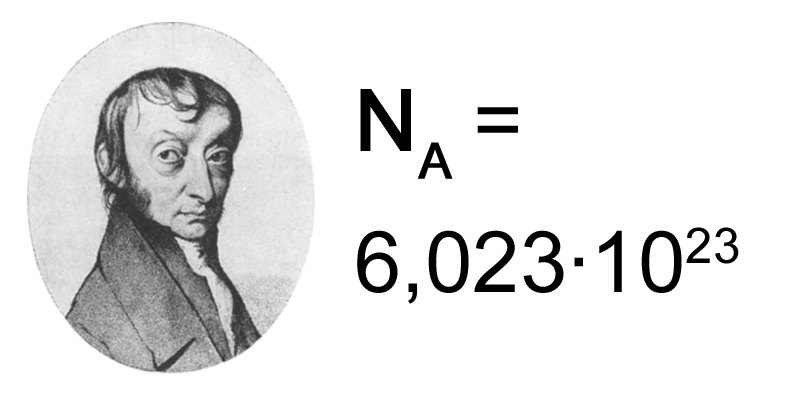The terms, mole, Avogadro’s number and Avogadro’s constant are related to each other. A mole is a unit used to measure the amount of a substance. It can be used to measure any type of chemical substance. The value of one mole is 6.022 x 1023. This is called the Avogadro’s number.  Avogadro’s number is the number of particles present in one mole of a substance. The mole of any substance is equal to this number of particles. These particles can be atoms, molecules, colloids or anything. Therefore, it is a constant number that is independent of the type of substance that is considered and is called the Avogadro’s constant. The main difference between Avogadro’s number and Avogadro’s constant is that Avogadro’s number is given as a number that has no units whereas the Avogadro’s constant is given in the unit of per mole (mol-1).

### Key Areas Covered

– Definition, Historical Background, Units, Applications
– Definition, Historical Background, Applications
– Comparison of Key Differences

Key Terms: Avogadro, Avogadro’s Constant, Avogadro’s Number, MoleAvogadro’s constant is the number of particles present in one mole of a substance. This is named after the scientist Amedeo Avogadro. This Avogadro’s constant was invented because scientists wanted to relate the amount of microscopic matter to the amount of macroscopic matter. For example, in a particular chemical reaction, the amount of a substance used should be related to the number of atoms or molecules that is present in that substance. Only then can we identify and predict the amount of product that is going to be given by that reaction.

The value of this constant was first calculated by the German scientist Jean-Baptiste Perrin. The value given for the Avogadro’s constant was 6.022 x 1023. This number was given a name “mole”. One mole of a substance is composed of 6.022 x 1023particles. For example, one mole of Sodium is composed of 6.022 x 1023 of sodium atoms. One mole of sodium chloride is composed of 6.022 x 1023of sodium chloride molecules. Therefore, the unit for Avogadro’s constant is mol-1. The Avogadro’s constant for one mole is 6.022 x 1023mol-1.

The Avogadro’s constant is useful in determining the amount of a substance that reacts in a particular chemical reaction. It is also helpful in determining the amount of product that is given by that reaction. Moreover, we can also predict whether that reaction would happen or not. The Avogadro’s constant is used in determining the atomic mass, molecular mass, and other related concepts.Avogadro’s number is 6.022 x 1023. It is the value given for the Avogadro’s Constant. The same thing represented by the Avogadro’s number was first calculated by a German scientist Johann Loschmidt but in different units. However, the real Avogadro’s number was calculated by the scientist Jean-Baptiste Perrin who gave this value a name Avogadro’s number in honor of Avogadro who first gave the concept about the mole. Later, the concepts regarding Avogadro’s number developed rapidly with the development of the science and technology.

One mole of a substance is composed of an Avogadro’s number of particles. These particles can be atoms, ions, molecules, colloids, etc. The particle that is being considered depends on the nature of the substance that is being considered. This term is useful in determining the atomic masses and molecular masses since Avogadro’s number indicates the number of atoms present in one mole of a chemical element or the number of molecules present in a particular compound.

### Definition

Avogadro’s Constant: Avogadro’s constant is the number of particles present in one mole of a substance.

### Other Definitions

Avogadro’s Constant: Avogadro’s constant states that the number of basic units present in a mole of a particular compound is a constant value for any compound.

Avogadro’s Number: Avogadro’s number is the number of hydrogen atoms present in one gram of 1H or number of carbon atoms present in one gram of 12C sample.

### Conclusion

Both Avogadro’s number and Avogadro’s constant describes the same concept. Both these concepts describe the number of particles present in one mole of a particular substance. They have the same value. However, they differ from each other according to their units. The main difference between Avogadro’s number and the Avogadro’s constant is that Avogadro’s number is given as a number that has no units whereas the Avogadro’s constant is given in the unit of per mole (mol-1).

##### References:

1. “The Mole and Avogadro’s Constant.” Chemistry LibreTexts, Libretexts, 21 July 2016, Available here. Accessed 18 Sept. 2017.
2. “Avogadro constant.” Wikipedia, Wikimedia Foundation, 10 Sept. 2017, Available here. Accessed 18 Sept. 2017.
3. The Editors of Encyclopædia Britannica. “Avogadro’s number.” Encyclopædia Britannica, Encyclopædia Britannica, inc., 10 June 2016, Available here. Accessed 18 Sept. 2017.

##### Image Courtesy:

1.”Nombre avogadro” By From Joanjoc – Done by myself using PD image; Image:Amedeo Avogadro.gif (Public Domain) via Commons Wikimedia